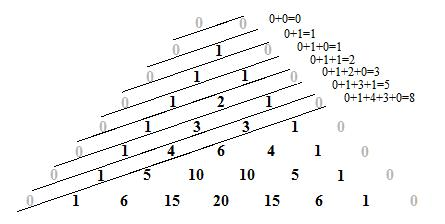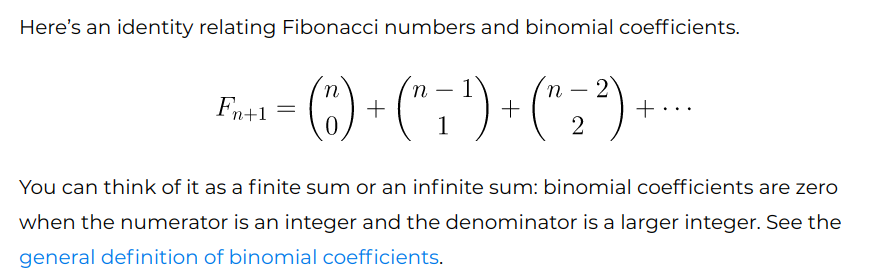# An interesting observation

• So from the simpler problem method (Fibonacci) and the casework method, can't we form a relation between the Fibonacci numbers?

• Hi @professionalbronco! Sorry, I'm not sure I completely understand your question... The Fibonacci numbers are defined as the sequence of numbers that begins with 1, 1, and then each number afterwards is the sum of the previous two numbers-- or in "math terms," a_(n+1) = a_(n) + a_(n-1). (In the block problem, we saw that the relationship between # ways to tile a 1xn area with 1x1 and 1x2 rectangles actually follows this Fibonacci pattern as well) If you mean an explicit formula to figure out, say, the 14th Fibonacci number without knowing the 12th and 13th, that does exist, although it's a bit complicated• @audrey Sorry if I didn't make my question clear, I meant from this problem, is there a way to relate binomial coefficients to Fibonacci numbers? Is there a formula to get $$F_n$$ using binomial coefficients?

• @professionalbronco Ah, thanks for the clarification! I'm pretty sure there is not a way to get F_n using binomial coefficients (if one of the other mods knows of one, though, they can respond :D). EDIT: my bad, turns out there is a connection- thanks @quacker88 !

And there is another way to figure out the Fibonacci number F_n explicitly (I think you can search "Fibonacci rule explicit form" or something)-- it doesn't have to do with binomial coefficients and it's a bit complicated, but you essentially make an educated "guess" for the formula's form, and then use the recursive form to solve for the explicit form.• @professionalbronco @audrey this is really interesting! I did some digging online and it turns out there actually is a relationship between Pascal's Triangle and the Fibonacci numbers! A lot of it is pretty complicated, but here's a beautiful image that about sums it all up:Summing along the diagonals actually gives you the Fibonacci numbers.

In an actual equation, it looks like**what the caption is saying is that if the denominator is larger than the numerator, by default the answer will be $$0$$. for example, look at "$$3$$ choose $$9$$" $$\binom{3}{9}$$: you can't really choose $$9$$ things from $$3$$ things, so the answer is just $$0$$. So, the sum looks like it goes on forever but eventually it will just be adding a bunch of $$0$$s.

Anyways, on that same website (it's the second link I have below) there was actually a very interesting proof that uses what @audrey brought up about the $$1\text{ x n}$$ tiles.

For example, let's think about $$F_8$$, which is the number of ways we can tile a $$1\text{ x 8}$$ strip with only $$1\text{ x 1}$$ and $$1\text{ x 2}$$ tiles. One way, like we saw earlier, to do it is to add $$F_7+F_6$$. Another way to think about it though, is to do it by casework.

If we have $$8$$ $$1\text{ x 1}$$ tiles and $$0$$ $$1\text{ x 2}$$ tiles, there is $$\binom80=$$$$1$$ way to tile the floor.

If we have $$6$$ $$1\text{ x 1}$$ tiles and $$1$$ $$1\text{ x 2}$$ tiles, there are $$\binom71=7$$ ways to tile the floor. (think about it like we're choosing a place to put the $$1\text{ x 2 }$$tile and then filling the rest with the remaining $$1\text {x 1 }$$s

If we have $$4$$ $$1\text{ x 1}$$ tiles and $$2$$ $$1\text{ x 2}$$ tiles, there are $$\binom62=15$$ ways to tile the floor.

This pattern is exactly what the equation described: after going all the way down we'll have that $$F_8=\binom80 + \binom71 + \binom62 + \binom53 +\binom44=34$$.

**34 is actually $$F_{9}$$ because the tile method has $$F_0 = 1, F_1=1, F_2=2$$ while the sequence actually starts on $$F_0=0, F_1=1, F_2=1$$

Hope this made senseThese were the sources I used:
StackExchange
John D. Cook

If you guys want to check them out, go ahead! But some of the stuff is quite advanced, I wasn't able to catch a lot of it either. Really interesting stuff though. Great observation, I learned a lot thanks to @professionalbronco bringing it up. Hope you guys did too!

• @audrey @quacker88 Thank you! I actually knew the way to generate the Fibonacci Numbers from Pascal's Triangle, I just didn't know how to write it with combinations. So for the

$$F_{n+1}=\binom{n}{0}+\binom{n-1}{1}+\binom{n-2}{2}+\cdots$$

I have two questions:

1. Where do we stop at? Do we stop when the binomial coefficient equals $$1?$$
2. Since we know that the above is true, then wouldn't the $$n$$th Fibonacci Number be
$$\binom{n-1}{0}+\binom{n-2}{1}+\binom{n-3}{2}+\cdots$$

?
Also, did you mean $$\binom{8}{0}=1$$?

• Hey @professionalbronco, good points you bring up:

Actually, for the combinations, you can look at Pascal's triangle and trace it out. For the example from earlier, recall that $$\binom80$$ is the $$0$$th number in the $$8$$th row. Doing this for each combination, you can see that it traces out a diagonal. That's probably the thought process I would use for converting the Pascal's Triangle into combinations

1. Yeah! After it equals $$1$$ again, every combination after that equals $$0$$, because if you have a combination where the numerator is smaller than the denominator, it equals $$0$$ (this goes back to the 3 choose 9 example I was talking about. It doesn't make sense, right?)
2. Right! Exactly. That's why the example I used was actually $$F_9$$ since the first combination was $$\binom80$$.

And yes, great catch. $$\binom80=1\neq0$$, I'll go and fix that nowthank you!!

Great work!